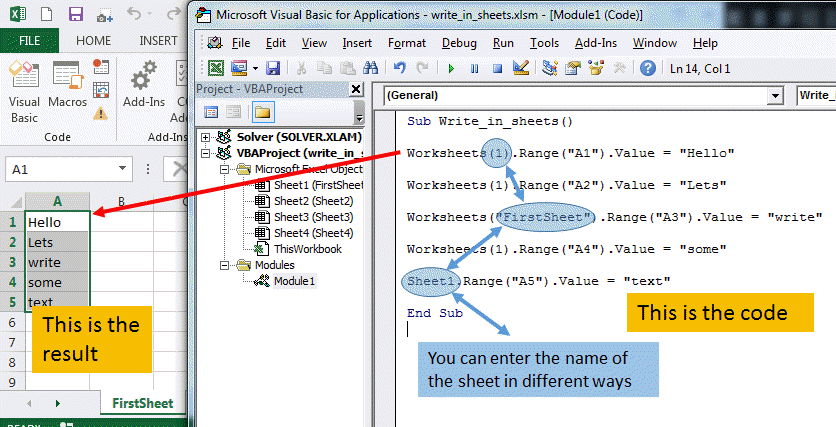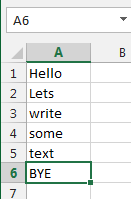## Write to cell and change worksheet values with VBA (and VBA object hierarchy)

Writing values in your Excel sheets with VBA can sometimes be a bit tricky. But it is essential for a correct understanding of VBA and the interaction between the user and the sheet. This interaction is done via OBJECTS.

This Objects are like grand-parents, parents and children . There is an order to all this.

The Object on the top, the common ancester, the great great great grand father or mother is Excel itself. It is called Application.

Then comes the Workbooks: this is the name of your file like "Myfile.xlsx" or "Myfile.xlsm" where m stands for macro.

Then comes the Worksheets which are the different sheets you have in your Workbook.

Then comes the Range which defines the cells that we are interested in.

And then comes the Attribute to the cell.

In VBA you can write a value in cell like in that case we give the cell A1 the value of "excel made easy".

This was it for a short introduction to hierarchies of Objects in Excel.

Now lets look at this in more details and how to write to a cell with VBA.

To write a variable to a cell from VBA, you have to know in which worksheet the cell is located. Is it the sheet1 or sheet2, ....?

So to enter a value in a cell use following command line.

Worksheets(1).Range("A1").Value = "Hello"

Where Worksheet(1) refers to the first worksheet. You can see, this is referred with a number 1, before we used its name "Sheet 1".

Here an example on how to write in the sheet in different manners using VBA.You can see that there are many ways to write text in a sheet.

Worksheets(1).Range("A1").Value = "Hello"

Worksheets(1).Range("A2").Value = "Lets"

Worksheets("FirstSheet").Range("A3").Value = "write"

Worksheets(1).Range("A4").Value = "some"

Sheet1.Range("A5").Value = "text"

### TIP:

It is important to understand the principle of hierarchy in Excel or any MS Office application.

In the following line, we write in the sheet1, in cell A6 the value BYE.

Application.Workbooks("write_in_sheets.xlsm").Worksheets(1).Range("A6").Value = "BYE"To write a variable to a cell in Excel with VBA, write following VBA code.

Application.Workbooks("write_in_sheets.xlsm").Worksheets(1).Range("A6").Value = MyVariable

Where MyVariable contains the value you want to write to the Cell

Application is the top hierarchical level.

Workbook(name of the file) will specify which file you are working in.

The next part Worksheet(1),Range() are explained before.

### Add a workbook with VBA

This will create a new workbook.

### Add a worksheet in Excel with VBA

This will add a worksheet in you workbook.

### Count the worksheets in VBA

This will return the number of worksheet in you workbook.

MsgBox Worksheets.Count   will display the number of worksheets.

a= Worksheets.Count    will give to the value a the number of worksheets.

Workbooks.Count will count the number of workbooks

the Count property of the Workbooks collection counts the number of active workbooks.

Please Tweet, Like or Share us if you enjoyed.